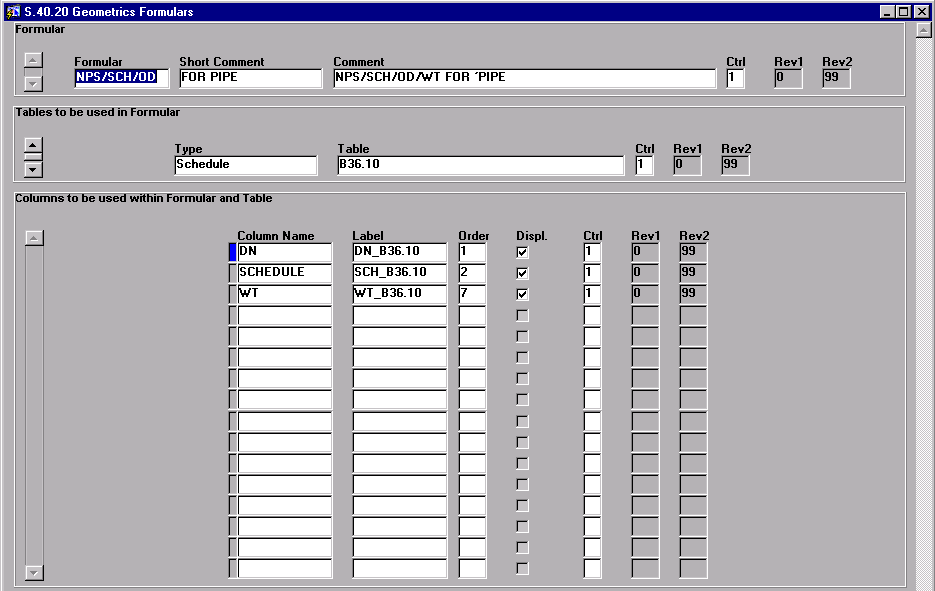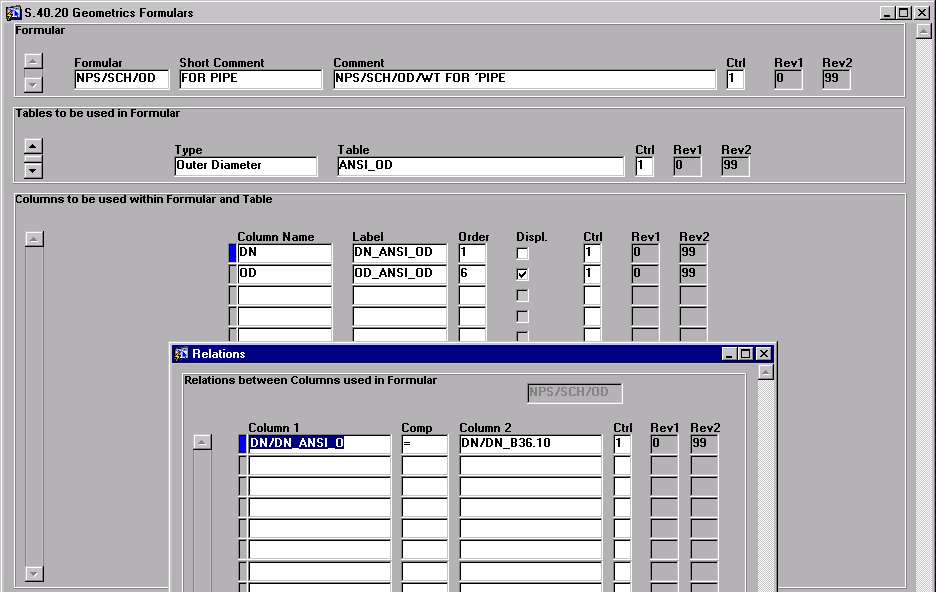# S.40.20 Geometric Formulas - Intergraph Smart Reference Data - Help - Hexagon PPM

## Intergraph Smart Reference Data Help Classic (2020)

Language
English (United States)
Product
Intergraph Smart Reference Data
Search by Category
Help
Smart Materials/Smart Reference Data Version
2020 (10.0)

The S.40.20 Geometric Formulas screen allows you to define the standard tables that are used to copy the required values into the new user-defined target table.First, generate a relation between the applied standard tables, as explained in the following procedure.

As a prerequisite, you must know in which tables the required values are stored. Then proceed as follows:

1. In the first (Formular) block, type the formular name in the Formular field, and the description in the Short Comment and Comment fields.

2. Save the formular.

3. In the Type field, select the type of the first standard geometric to be used to copy values from.

4. In the Table field, select the name of the geometric table that contains the required data.

5. Click Save.

6. In the third block, use the LOV in the Column Name field to select the table columns.

7. Type the text for the column label in the Label field,

8. In the Order field, assign a numeric value to the selected column according to the Physical Attribute definition made on the A.50.03 Tables/Attributes screen.

For example, the DN attribute is assigned to the first input colum, and the OD attribute is assigned to the first output column (which is the 6th table column), you enter 1 in the Order field for DN, and 6 for OD.

9. Select Displ. if the value should be displayed in the target geometric table.

10. Repeat the previous steps for all geometric tables and columns that are needed for the geometric simulation.

11. After saving, click Next Block to display the Relations screen.12. If you use more than one standard table to provide your values, you must create a relation between the tables. For example, if you use two different tables containing two sets of values to produce your target table, and both tables contain the same value such as DN, then DN becomes the relation link between both tables.

In another example, the column names NPS, SCH, OD and WT are required to produce the user-defined target table. Two standard geometrics are necessary to provide all needed data. The first geometric contains the NPS, SCH and WT values, and the second geometric the OD and NPS values. In this example, the NPS value is the connecting link between both tables. The relation definition would be as follows:

NPS/table1 = NPS/table2

For Table1/2, the LABEL wording must be used.